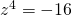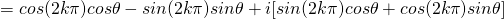# Solving roots of higher orderThis is one question that I know ALL my students can excel in doing, that is solve. So I’m not interested in showing you how to solve such problems, but I want to explain a particular step which you introduce in your working. So let’s take a quick look at the solution first.forSo here, we note that we introduced, but how is?

Intuitively,foris simply full circle. So we are really just turning full circles about the same point here, which is why we are still referring to the same number.

I will do a simple mathematical proof here too.(using formulas in th MF15)

ForNot readable? Change text.